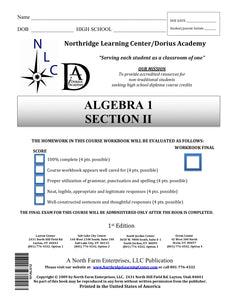Algebra I, Section II

Regular price
\$56.50
Regular price
Sale price
\$56.50
Unit price
per
Availability
Sold out

Algebra I 07-02-00-00-010

In this course, students will learn the fundamental concepts of algebra they will need to continue their math education. Beginning with the introduction of mathematical variables and progressing through solving, graphing, and interpreting basic linear equations, this course prepares students for both Geometry and Algebra II. At the end of this course, students will be expected to show mastery of techniques such as order of operations, factoring, distribution, substitution, elimination, solving equations, and graphing both equations and inequalities.